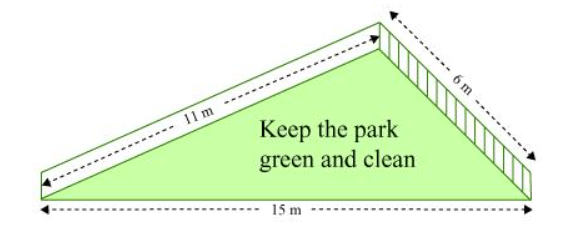# There is a slide in the park.

Question. There is a slide in the park. One of its side walls has been painted in the same colour with a message “KEEP THE PARK GREEN AND CLEAN” (see the given figure). If the sides of the wall are 15m, 11m, and 6m, find the area painted in colour.Solution:

t can be observed that the area to be painted in colour is a triangle, having its sides as 11 m, 6 m, and 15 m.

Perimeter of such a triangle $=(11+6+15) \mathrm{m}$

$2 s=32 \mathrm{~m}$

$s=16 \mathrm{~m}$

By Heron's formula,

Area of triangle $=\sqrt{s(s-a)(s-b)(s-c)}$

$=[\sqrt{16(16-11)(16-6)(16-15)}] \mathrm{m}^{2}$

$=(\sqrt{16 \times 5 \times 10 \times 1}) \mathrm{m}^{2}$

$=20 \sqrt{2} \mathrm{~m}^{2}$

Therefore, the area painted in colour is $20 \sqrt{2} \mathrm{~m}^{2}$.